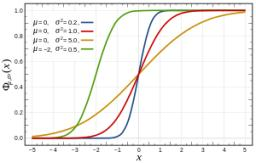# Distribution 4907

A continuous random variable X is specified: distribution function, specify the parameters a; b so that the function F (x) is continuous and was the distribution function of the random variable X and express f(x).
P (X <5)
F (x) = 0; x <3
F (x) = a. x - b; 3 F (x) = 1; 6> x

a =  0.3333
b =  1

### Step-by-step explanation:

0=a·3-b
1 = a·6-b

3a-b = 0
6a-b = 1

Pivot: Row 1 ↔ Row 2
6a-b = 1
3a-b = 0

Row 2 - 3/6 · Row 1 → Row 2
6a-b = 1
-0.5b = -0.5

b = -0.5/-0.5 = 1
a = 1+b/6 = 1+1/6 = 0.33333333

a = 1/3 ≈ 0.333333
b = 1

Our linear equations calculator calculates it.Did you find an error or inaccuracy? Feel free to write us. Thank you!

Tips for related online calculators
Do you have a linear equation or system of equations and looking for its solution? Or do you have a quadratic equation?
Would you like to compute the count of combinations?